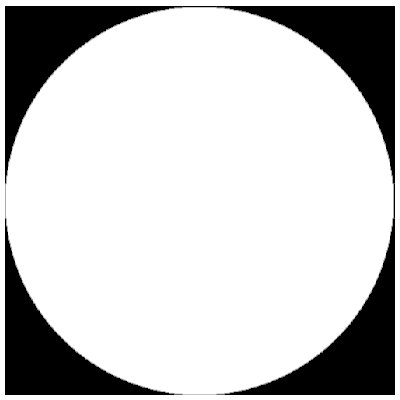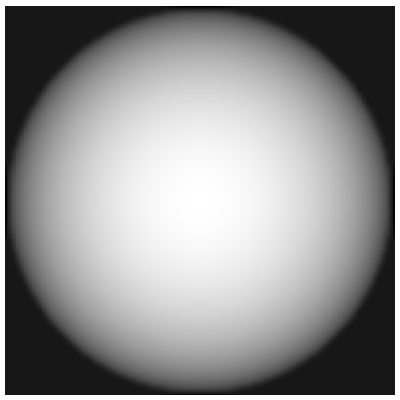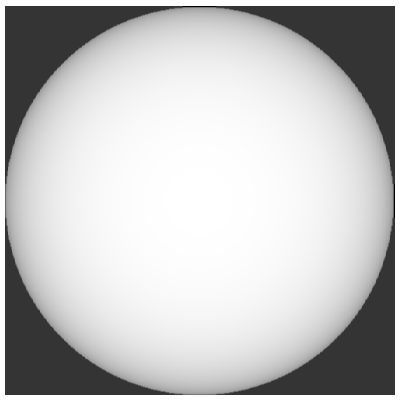The other day, I was wondering: what does a sphere look like that is a Lambertian reflector? And is a Lambertian model valid for a celestial body?

Obviously, a celestial body is not mirror smooth, so its surface is not specular. And as far as I used to know, if something is not specular, it is diffuse, i.e., Lambertian. The difference is that a specular surface reflects light as a mirror; a Lambertian surface reflects light in all directions, regardless of the angle of incidence.

But then... what about a surface made of corner cubes, like a cat's retina? It is obviously not specular... but it doesn't exactly obey Lambert's cosine law either.

But I am jumping ahead of myself.

Lambert's cosine law says that the intensity of light emitted by a Lambertian surface element varies as $\cos\theta,$ where $\theta$ is the angle of the ray of light with respect to the normal of that surface element.

So let us first consider a sphere that is a uniform source of light. Each surface element will be emitting light with an intensity proportional to $\cos\theta.$ But each surface element will be seen under an angle $\cos\theta$, its apparent cross-section reduced. So the actual intensity will be given by $\cos\theta/\cos\theta=1,$ i.e., it will be uniform:Terribly boring, isn't it.

Next, let us consider a sphere that is a Lambertian reflector, illuminated from the viewing direction. The amount of illumination falling onto a surface element will be proportional to $\cos\theta=\sqrt{1-r^2},$ where $r$ is the projected radius (normalized to 1) of the disk that we see. This will be multiplied, as before, by $\cos\theta/\cos\theta=1,$ to account for the Lambertian nature of the surface and for the viewing angle. In short, the brightness of the disk will be proportional to $\sqrt{1-r^2}$:Which of these is more like the Moon, though? Of course the Moon has surface features, as its surface is not perfectly uniform, dotted as it is with craters, marina, mountains and valleys. But does it fade near the edges of the lunar disk?No, it does not appear so. How is this possible?

To understand why, I searched the literature. Eventually, I happened upon a paper  by Michael Oren and Shree K. Nayar, from 1995. Their model takes into account something that is not properly captured by either the Lambertian or the specular model: surface roughness.

Contrary to naive expectations, a rough surface does not act like a Lambertian reflector. Its behavior is closer (though not identical) to that of a retroreflector, which reflects light preferentially in the direction from which the light comes.

Oren and Nayar work out a generalization of the Lambertian model that takes surface roughness into account, characterizing it by a standard deviation parameter $\sigma.$ The resulting model is given by

$$L\propto \cos\theta_i\cdot\left[1-0.5\frac{\sigma^2}{\sigma^2+0.33}+0.45\frac{\sigma^2}{\sigma^2+0.09}\sin\theta_i\sin\theta_r\frac{{\rm max}(0,\cos\phi)}{\cos\left({\rm max}(\theta_i,\theta_r)\right)}\right],$$

where $\theta_i$ and $\theta_r$ are the angles of incidence and reflectance, respectively, and $\phi$ is the azimuthal angle between the incident and reflected ray of light.

In the specific case when the illumination is from the viewing direction, we have $\theta_i=\theta_r=\arcsin r$ and $\phi=0$, hence

$$L\propto \sqrt{1-r^2}\cdot\left[1-0.5\frac{\sigma^2}{\sigma^2+0.33}+0.45\frac{\sigma^2}{\sigma^2+0.09}\frac{r^2}{\sqrt{1-r^2}}\right].$$

For $\sigma=0.5$, we get this result:This is a lot more like the lunar disk, isn't it!

OK, so the Moon has surface roughness. The Sun, on the other hand, does not. And in any case, it is not a reflecting surface, but a light emitting one. So it really should appear like a flat disk. Does it? Well... not exactly:(Ignore the transiting Venus in the upper right corner, by the way.) Clearly, the solar disk is dimmer near the edges. How can that be?

This phenomenon, called limb darkening, has a completely different explanation. It has to do with the fact that above the visible, light-emitting surface of the Sun there is a thick, mostly transparent atmosphere. Mostly, but not completely transparent. So light coming from near the edges of the solar disk, which travel at a shallow angle relative to the solar surface, travel a much greater distance in the solar atmosphere than light coming from the middle of the solar disk, in a direction approximately perpendicular to the solar surface. The greater the distance, the more light is absorbed by the solar atmosphere, which explains why light from the limb appears dimmer.

I find this situation sweetly ironic, though, because in the end, we find that the Sun (supposedly a Lambertian emitter) behaves like the Moon should if it were a Lambertian reflector; and the Moon (supposedly a Lambertian reflector) behaves like the Sun should if it had no atmosphere.

It's almost as though Nature was trying to purposefully confuse us.

 Oren, M. & Nayar, S.K. Int J Comput Vision (1995) 14: 227. https://doi.org/10.1007/BF01679684